QMargins

QLine

# QLineF¶

## Detailed Description¶

The PySide.QtCore.QLineF class provides a two-dimensional vector using floating point precision.

A PySide.QtCore.QLineF describes a finite length line (or line segment) on a two-dimensional surface. PySide.QtCore.QLineF defines the start and end points of the line using floating point accuracy for coordinates. Use the PySide.QtCore.QLineF.toLine() function to retrieve an integer based copy of this line.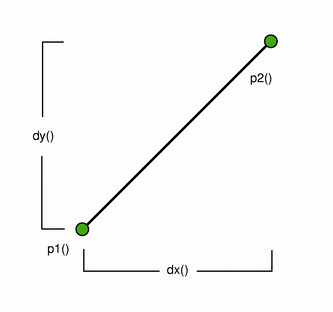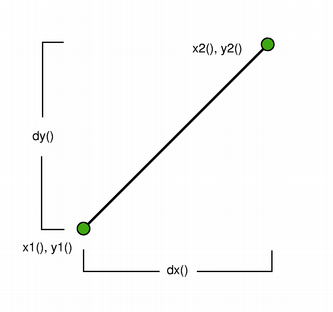The positions of the line’s start and end points can be retrieved using the PySide.QtCore.QLineF.p1() , PySide.QtCore.QLineF.x1() , PySide.QtCore.QLineF.y1() , PySide.QtCore.QLineF.p2() , PySide.QtCore.QLineF.x2() , and PySide.QtCore.QLineF.y2() functions. The PySide.QtCore.QLineF.dx() and PySide.QtCore.QLineF.dy() functions return the horizontal and vertical components of the line, respectively.

The line’s length can be retrieved using the PySide.QtCore.QLineF.length() function, and altered using the PySide.QtCore.QLineF.setLength() function. Similarly, PySide.QtCore.QLineF.angle() and PySide.QtCore.QLineF.setAngle() are respectively used for retrieving and altering the angle of the line. Use the PySide.QtCore.QLineF.isNull() function to determine whether the PySide.QtCore.QLineF represents a valid line or a null line.

The PySide.QtCore.QLineF.intersect() function determines the QLineF.IntersectType for this line and a given line, while the PySide.QtCore.QLineF.angle() function returns the angle between the lines. In addition, the PySide.QtCore.QLineF.unitVector() function returns a line that has the same starting point as this line, but with a length of only 1, while the PySide.QtCore.QLineF.normalVector() function returns a line that is perpendicular to this line with the same starting point and length.

Finally, the line can be translated a given offset using the PySide.QtCore.QLineF.translate() function, and can be traversed using the PySide.QtCore.QLineF.pointAt() function.

class PySide.QtCore.QLineF
class PySide.QtCore.QLineF(line)
class PySide.QtCore.QLineF(QLineF)
class PySide.QtCore.QLineF(pt1, pt2)
class PySide.QtCore.QLineF(x1, y1, x2, y2)
Parameters: QLineF – PySide.QtCore.QLineF line – PySide.QtCore.QLine pt1 – PySide.QtCore.QPointF pt2 – PySide.QtCore.QPointF y1 – PySide.QtCore.qreal x1 – PySide.QtCore.qreal y2 – PySide.QtCore.qreal x2 – PySide.QtCore.qreal

Constructs a null line.

Construct a PySide.QtCore.QLineF object from the given integer-based line .

Constructs a line object that represents the line between p1 and p2 .

Constructs a line object that represents the line between (x1 , y1 ) and (x2 , y2 ).

PySide.QtCore.QLineF.IntersectType

Describes the intersection between two lines.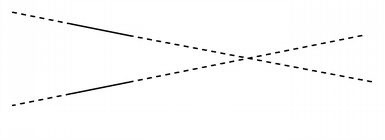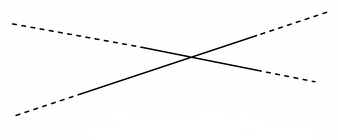QLineF.UnboundedIntersection QLineF.BoundedIntersection
Constant Description
QLineF.NoIntersection Indicates that the lines do not intersect; i.e. they are parallel.
QLineF.UnboundedIntersection The two lines intersect, but not within the range defined by their lengths. This will be the case if the lines are not parallel.

PySide.QtCore.QLineF.intersect() will also return this value if the intersect point is within the start and end point of only one of the lines.

Constant Description
QLineF.BoundedIntersection The two lines intersect with each other within the start and end points of each line.
PySide.QtCore.QLineF.__reduce__()
Return type: PyObject
PySide.QtCore.QLineF.__repr__()
Return type: PyObject
PySide.QtCore.QLineF.angle(l)
Parameters: l – PySide.QtCore.QLineF PySide.QtCore.qreal

Returns the angle (in degrees) between this line and the given line , taking the direction of the lines into account. If the lines do not intersect within their range, it is the intersection point of the extended lines that serves as origin (see QLineF.UnboundedIntersection ).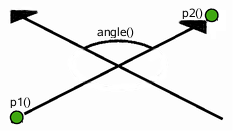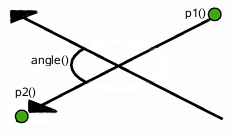When the lines are parallel, this function returns 0 if they have the same direction; otherwise it returns 180.

PySide.QtCore.QLineF.angle()
Return type: PySide.QtCore.qreal

Returns the angle of the line in degrees.

The return value will be in the range of values from 0.0 up to but not including 360.0. The angles are measured counter-clockwise from a point on the x-axis to the right of the origin (x > 0).

PySide.QtCore.QLineF.angleTo(l)
Parameters: l – PySide.QtCore.QLineF PySide.QtCore.qreal

Returns the angle (in positive degrees) from this line to the given line , taking the direction of the lines into account. If the lines do not intersect within their range, it is the intersection point of the extended lines that serves as origin (see QLineF.UnboundedIntersection ).

The returned value represents the number of degrees you need to add to this line to make it have the same angle as the given line , going counter-clockwise.

PySide.QtCore.QLineF.dx()
Return type: PySide.QtCore.qreal

Returns the horizontal component of the line’s vector. Return value is positive if PySide.QtCore.QLineF.x2() >= PySide.QtCore.QLineF.x1() and negative if PySide.QtCore.QLineF.x2() < PySide.QtCore.QLineF.x1() .

PySide.QtCore.QLineF.dy()
Return type: PySide.QtCore.qreal

Returns the vertical component of the line’s vector. Return value is positive if PySide.QtCore.QLineF.y2() >= PySide.QtCore.QLineF.y1() and negative if PySide.QtCore.QLineF.y2() < PySide.QtCore.QLineF.y1() .

static PySide.QtCore.QLineF.fromPolar(length, angle)
Parameters: length – PySide.QtCore.qreal angle – PySide.QtCore.qreal PySide.QtCore.QLineF

Returns a PySide.QtCore.QLineF with the given length and angle .

The first point of the line will be on the origin.

Positive values for the angles mean counter-clockwise while negative values mean the clockwise direction. Zero degrees is at the 3 o’clock position.

PySide.QtCore.QLineF.intersect(l)
Parameters: l – PySide.QtCore.QLineF (intersectType, intersectionPoint)

Returns a value indicating whether or not this line intersects with the given line .

The actual intersection point is extracted to intersectionPoint (if the pointer is valid). If the lines are parallel, the intersection point is undefined.

PySide.QtCore.QLineF.isNull()
Return type: PySide.QtCore.bool

Returns true if the line is not set up with valid start and end point; otherwise returns false.

PySide.QtCore.QLineF.length()
Return type: PySide.QtCore.qreal

Returns the length of the line.

PySide.QtCore.QLineF.normalVector()
Return type: PySide.QtCore.QLineF

Returns a line that is perpendicular to this line with the same starting point and length.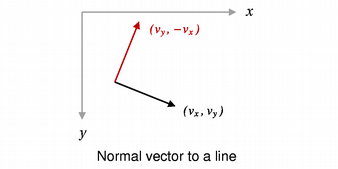PySide.QtCore.QLineF.__ne__(d)
Parameters: d – PySide.QtCore.QLineF PySide.QtCore.bool

Returns true if the given line is not the same as this line.

A line is different from another line if their start or end points differ, or the internal order of the points is different.

PySide.QtCore.QLineF.__mul__(m)
Parameters: m – PySide.QtGui.QMatrix PySide.QtCore.QLineF
PySide.QtCore.QLineF.__mul__(m)
Parameters: m – PySide.QtGui.QTransform PySide.QtCore.QLineF
PySide.QtCore.QLineF.__eq__(d)
Parameters: d – PySide.QtCore.QLineF PySide.QtCore.bool

Returns true if the given line is the same as this line.

A line is identical to another line if the start and end points are identical, and the internal order of the points is the same.

PySide.QtCore.QLineF.p1()
Return type: PySide.QtCore.QPointF

Returns the line’s start point.

PySide.QtCore.QLineF.p2()
Return type: PySide.QtCore.QPointF

Returns the line’s end point.

PySide.QtCore.QLineF.pointAt(t)
Parameters: t – PySide.QtCore.qreal PySide.QtCore.QPointF

Returns the point at the parameterized position specified by t . The function returns the line’s start point if t = 0, and its end point if t = 1.

PySide.QtCore.QLineF.setAngle(angle)
Parameters: angle – PySide.QtCore.qreal

Sets the angle of the line to the given angle (in degrees). This will change the position of the second point of the line such that the line has the given angle.

Positive values for the angles mean counter-clockwise while negative values mean the clockwise direction. Zero degrees is at the 3 o’clock position.

PySide.QtCore.QLineF.setLength(len)
Parameters: len – PySide.QtCore.qreal

Sets the length of the line to the given length . PySide.QtCore.QLineF will move the end point - PySide.QtCore.QLineF.p2() - of the line to give the line its new length. If the given length is negative the PySide.QtCore.QLineF.angle() is also changed.

If the line is a null line, the length will remain zero regardless of the length specified.

PySide.QtCore.QLineF.setLine(x1, y1, x2, y2)
Parameters: x1 – PySide.QtCore.qreal y1 – PySide.QtCore.qreal x2 – PySide.QtCore.qreal y2 – PySide.QtCore.qreal

Sets this line to the start in x1 , y1 and end in x2 , y2 .

PySide.QtCore.QLineF.setP1(p1)
Parameters: p1 – PySide.QtCore.QPointF

Sets the starting point of this line to p1 .

PySide.QtCore.QLineF.setP2(p2)
Parameters: p2 – PySide.QtCore.QPointF

Sets the end point of this line to p2 .

PySide.QtCore.QLineF.setPoints(p1, p2)

Sets the start point of this line to p1 and the end point of this line to p2 .

PySide.QtCore.QLineF.toLine()
Return type: PySide.QtCore.QLine

Returns an integer based copy of this line.

Note that the returned line’s start and end points are rounded to the nearest integer.

PySide.QtCore.QLineF.QLineF()

PySide.QtCore.QLineF.toTuple()
Return type: PyObject
PySide.QtCore.QLineF.translate(p)
Parameters: p – PySide.QtCore.QPointF

Translates this line by the given offset .

PySide.QtCore.QLineF.translate(dx, dy)
Parameters: dx – PySide.QtCore.qreal dy – PySide.QtCore.qreal

This is an overloaded function.

Translates this line the distance specified by dx and dy .

PySide.QtCore.QLineF.translated(p)
Parameters: p – PySide.QtCore.QPointF PySide.QtCore.QLineF

Returns this line translated by the given offset .

PySide.QtCore.QLineF.translated(dx, dy)
Parameters: dx – PySide.QtCore.qreal dy – PySide.QtCore.qreal PySide.QtCore.QLineF

This is an overloaded function.

Returns this line translated the distance specified by dx and dy .

PySide.QtCore.QLineF.unitVector()
Return type: PySide.QtCore.QLineF

Returns the unit vector for this line, i.e a line starting at the same point as this line with a length of 1.0.

PySide.QtCore.QLineF.x1()
Return type: PySide.QtCore.qreal

Returns the x-coordinate of the line’s start point.

PySide.QtCore.QLineF.x2()
Return type: PySide.QtCore.qreal

Returns the x-coordinate of the line’s end point.

PySide.QtCore.QLineF.y1()
Return type: PySide.QtCore.qreal

Returns the y-coordinate of the line’s start point.

PySide.QtCore.QLineF.y2()
Return type: PySide.QtCore.qreal

Returns the y-coordinate of the line’s end point.